# Widest Path Problem | Practical application of Dijkstra’s Algorithm

It is highly recommended to read Dijkstra’s algorithm using the Priority Queue first.

Widest Path Problem is a problem of finding a path between two vertices of the graph maximizing the weight of the minimum-weight edge in the path. See the below image to get the idea of the problem: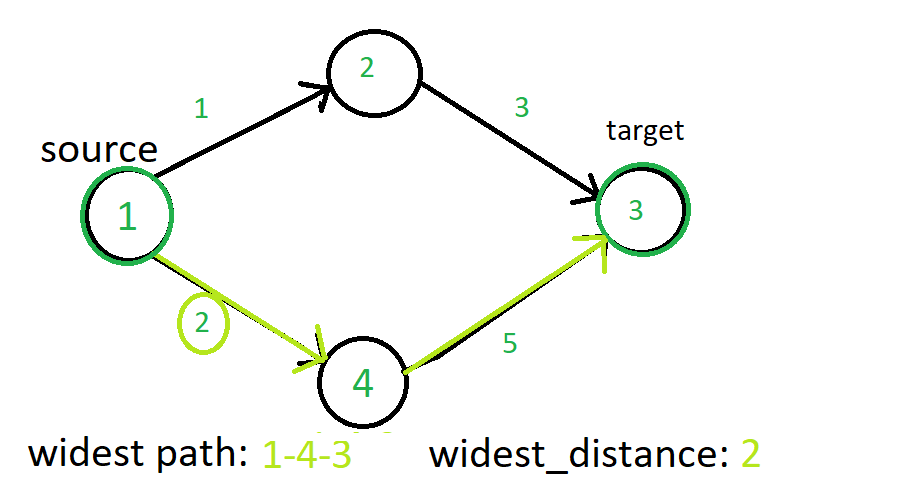Practical Application Example:
This problem is a famous variant of Dijkstra’s algorithm. In the practical application, this problem can be seen as a graph with routers as its vertices and edges represent bandwidth between two vertices. Now if we want to find the maximum bandwidth path between two places in the internet connection, then this problem can be solved by this algorithm.

How to solve this problem?
We are going to solve this problem by using the priority queue ((|E|+|V|)log|V|) implementation of the Dijkstra’s algorithm with a slight change.

We solve this problem by just replacing the condition of relaxation in Dijkstra’s algorithm by:

max(min(widest_dist[u], weight(u, v)), widest_dist[v])

where u is the source vertex for v. v is the current vertex we are checking the condition.

This algorithm runs for both directed and undirected graph.

See the series of images below to get the idea about the problem and the algorithm:

The values over the edges represents weights of directed edges.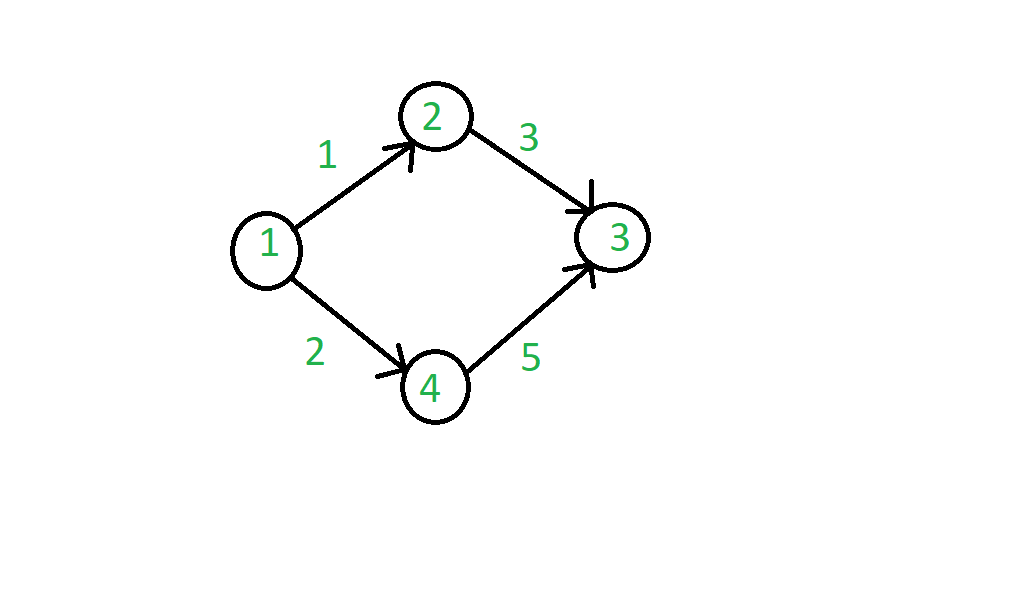We will start from source vertex and then travel all the vertex connected to it and add in priority queue according to relaxation condition.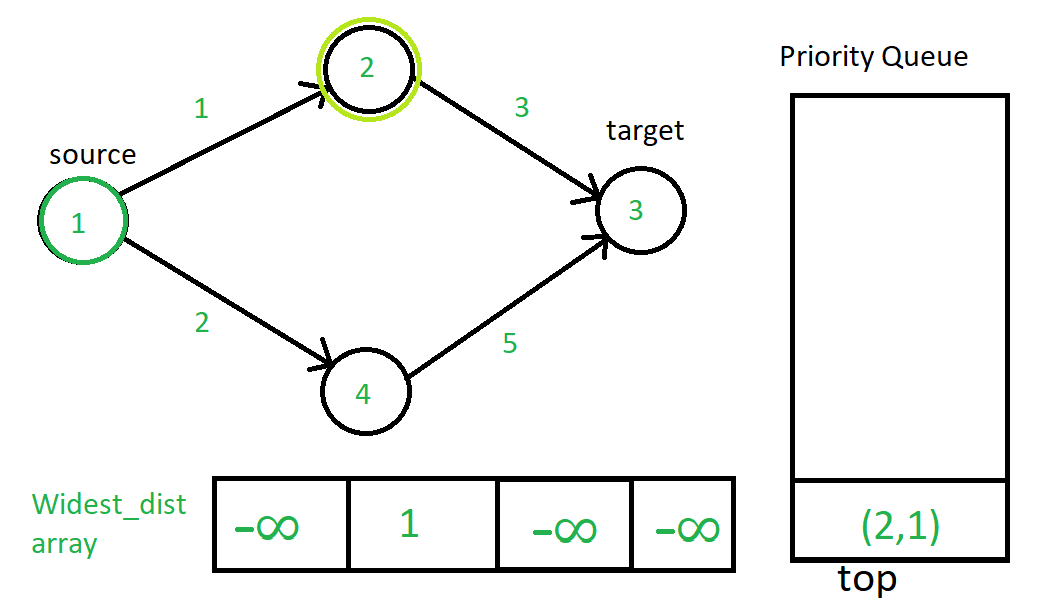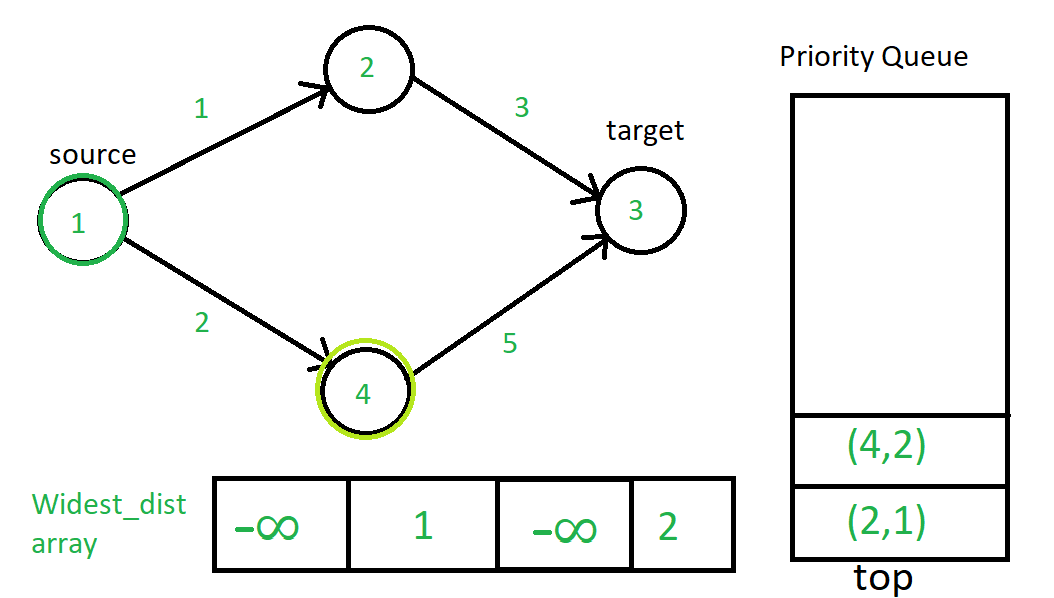Now (2, 1) will pop up and 2 will be the current source vertex.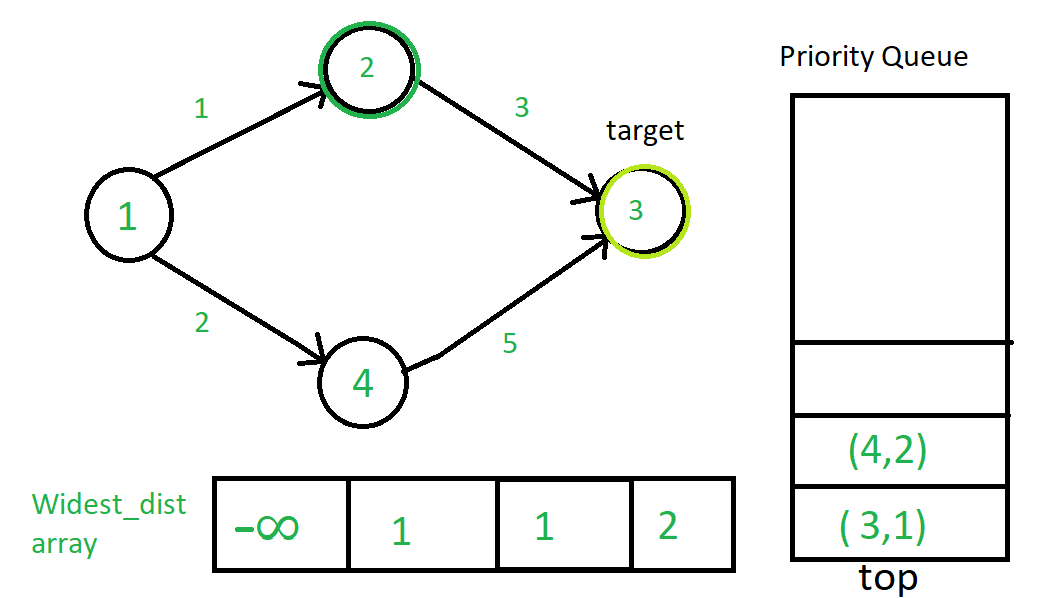Now (3, 1) will pop from the queue. But as 3 does not have any connected vertex through directed edge nothing will happen. So (4, 2) will pop next.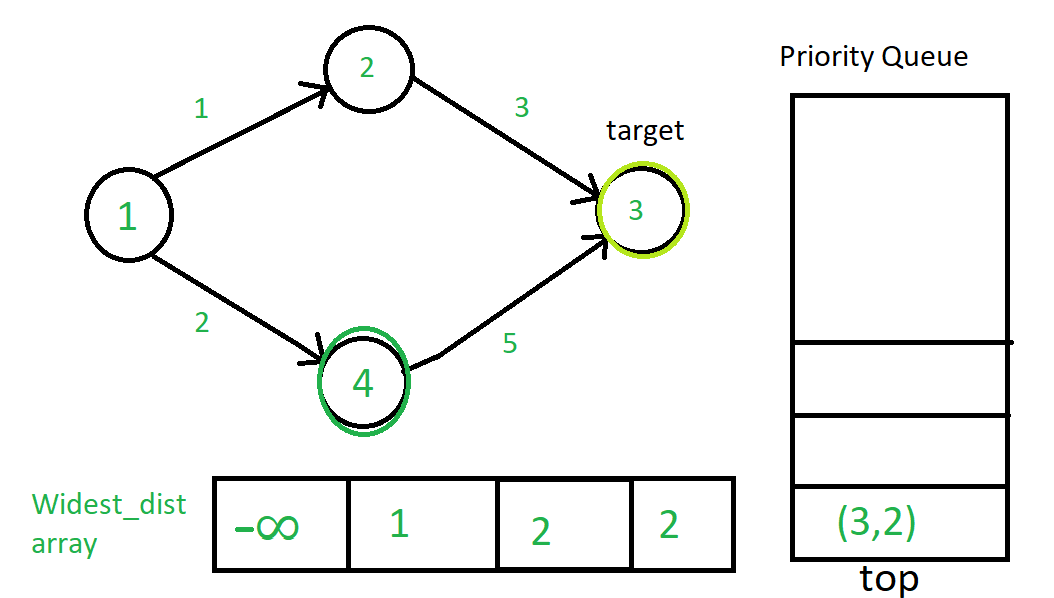Finally the algorithm stops, as there is no more elements in priority queue.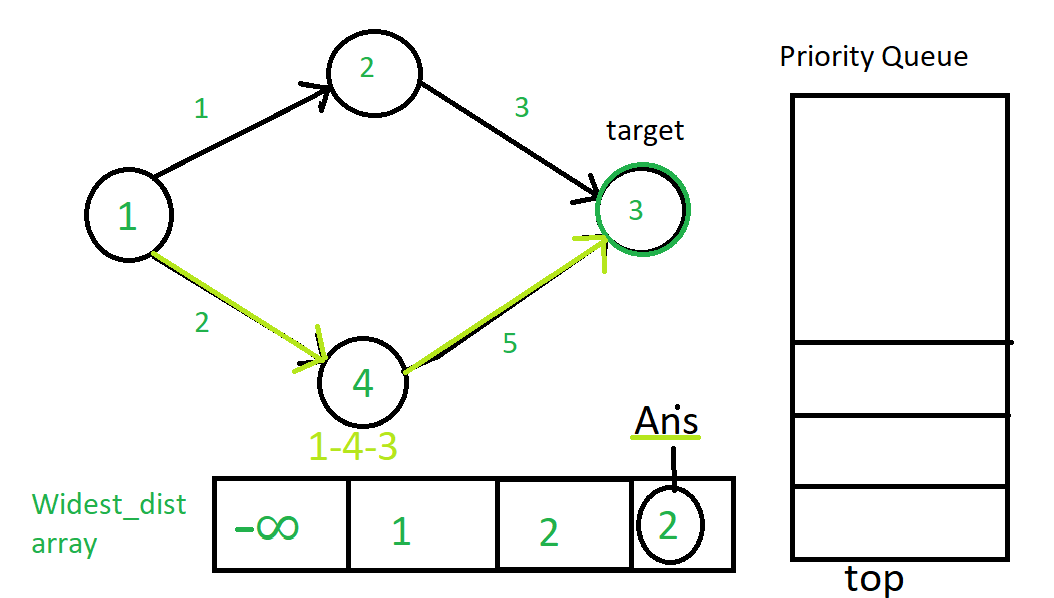The path with the maximum value of widest distance is 1-4-3 which has the maximum bottle-neck value of 2. So we end up getting widest distance of 2 to reach the target vertex 3.

Below is the implementation of the above approach:

 `// C++ implementation of the approach ` `#include ` `using` `namespace` `std; ` ` `  `// Function to print the required path ` `void` `printpath(vector<``int``>& parent, ``int` `vertex, ``int` `target) ` `{ ` `    ``if` `(vertex == 0) { ` `        ``return``; ` `    ``} ` ` `  `    ``printpath(parent, parent[vertex], target); ` ` `  `    ``cout << vertex << (vertex == target ? ``"\n"` `: ``"--"``); ` `} ` ` `  `// Function to return the maximum weight ` `// in the widest path of the given graph ` `int` `widest_path_problem(vector > >& Graph, ` `                        ``int` `src, ``int` `target) ` `{ ` `    ``// To keep track of widest distance ` `    ``vector<``int``> widest(Graph.size(), INT_MIN); ` ` `  `    ``// To get the path at the end of the algorithm ` `    ``vector<``int``> parent(Graph.size(), 0); ` ` `  `    ``// Use of Minimum Priority Queue to keep track minimum ` `    ``// widest distance vertex so far in the algorithm ` `    ``priority_queue, vector >, ` `                   ``greater > > ` `        ``container; ` ` `  `    ``container.push(make_pair(0, src)); ` ` `  `    ``widest[src] = INT_MAX; ` ` `  `    ``while` `(container.empty() == ``false``) { ` `        ``pair<``int``, ``int``> temp = container.top(); ` ` `  `        ``int` `current_src = temp.second; ` ` `  `        ``container.pop(); ` ` `  `        ``for` `(``auto` `vertex : Graph[current_src]) { ` ` `  `            ``// Finding the widest distance to the vertex ` `            ``// using current_source vertex's widest distance ` `            ``// and its widest distance so far ` `            ``int` `distance = max(widest[vertex.second], ` `                               ``min(widest[current_src], vertex.first)); ` ` `  `            ``// Relaxation of edge and adding into Priority Queue ` `            ``if` `(distance > widest[vertex.second]) { ` ` `  `                ``// Updating bottle-neck distance ` `                ``widest[vertex.second] = distance; ` ` `  `                ``// To keep track of parent ` `                ``parent[vertex.second] = current_src; ` ` `  `                ``// Adding the relaxed edge in the prority queue ` `                ``container.push(make_pair(distance, vertex.second)); ` `            ``} ` `        ``} ` `    ``} ` ` `  `    ``printpath(parent, target, target); ` ` `  `    ``return` `widest[target]; ` `} ` ` `  `// Driver code ` `int` `main() ` `{ ` ` `  `    ``// Graph representation ` `    ``vector > > graph; ` ` `  `    ``int` `no_vertices = 4; ` ` `  `    ``graph.assign(no_vertices + 1, vector >()); ` ` `  `    ``// Adding edges to graph ` ` `  `    ``// Resulting graph ` `    ``// 1--2 ` `    ``// |  | ` `    ``// 4--3 ` ` `  `    ``// Note that order in pair is (distance, vertex) ` `    ``graph.push_back(make_pair(1, 2)); ` `    ``graph.push_back(make_pair(2, 4)); ` `    ``graph.push_back(make_pair(3, 3)); ` `    ``graph.push_back(make_pair(5, 3)); ` ` `  `    ``cout << widest_path_problem(graph, 1, 3); ` ` `  `    ``return` `0; ` `} `

Output:

```1--4--3
2
```

GeeksforGeeks has prepared a complete interview preparation course with premium videos, theory, practice problems, TA support and many more features. Please refer Placement 100 for details

My Personal Notes arrow_drop_upCheck out this Author's contributed articles.

If you like GeeksforGeeks and would like to contribute, you can also write an article using contribute.geeksforgeeks.org or mail your article to contribute@geeksforgeeks.org. See your article appearing on the GeeksforGeeks main page and help other Geeks.

Please Improve this article if you find anything incorrect by clicking on the "Improve Article" button below.